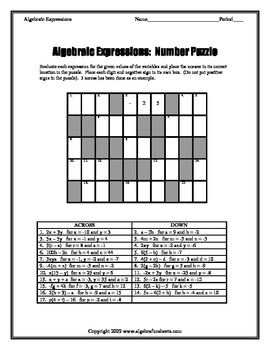Language of Algebra: Algebraic and Verbal Expressions (Bundle)7th - 11th
Subjects
Resource Type
Formats Included
• PDF
Pages
38 pages

Description

Bundle of 13 worksheets on algebraic, numerical and verbal expressions.

Worksheet 1: Algebraic Expressions Football

Standard. Given an expression for football scores, students will determine who wins and by how much. BASIC skills worksheet for students who need practice substituting values for variables.

Worksheet 2: Algebraic Expressions

SUDOKU. Positive integers only. Grouping symbols and exponents.

Worksheet 3: Algebraic Expressions

Riddle. Grouping symbols, no exponents. Problems have up to 5 different variables.

Worksheet 4: Algebraic Expressions

Number Puzzle. Grouping symbols, no exponents. Up to 3 variables, all integers.

Worksheet 5: Algebraic Expressions with Absolute Value

Standard. Students will evaluate single variable absolute value expressions.

Worksheet 6: Algebraic Expressions with Exponents

Standard. Focus on substituting negative values in exponential expressions. Up to 3 variable expressions.

Worksheet 7: Numerical Expressions. Adding and Subtracting Multiple Integers with Absolute Value

Standard. Multiple single digit integer addition/subtraction with absolute value.

Worksheet 8: Numerical Expressions

Riddle. Numerical expressions with exponents.

Worksheet 9: Order of Operations

Riddle. Integers and 4 basic math operations only. (No exponents or grouping symbols).

Worksheet 10: Verbal EQUATIONS

Standard. Multiple Choice. Find the algebraic equation given the verbal form.

Worksheet 11: Verbal Expressions 1

Riddle. Single operation word phrases only.

Worksheet 12: Verbal Expressions 2

Riddle. Up to two operations.

Worksheet 13: Verbal Expressions

Number Puzzle. Write algebraic expressions for given verbal expression.

Total of 38 pages since most funsheets have a standard worksheet version, which has the same problems, but gives options as to which format best suits your class. In addition, most answer keys are included for both funsheet and standard versions.

** Worksheets are copyright material and are intended for use in the classroom only. Purchased worksheets may NOT be posted on the internet, including but not limited to teacher web pages.**

Total Pages
38 pages
Included
Teaching Duration
N/A
Report this Resource to TpT
Reported resources will be reviewed by our team. Report this resource to let us know if this resource violates TpT’s content guidelines.